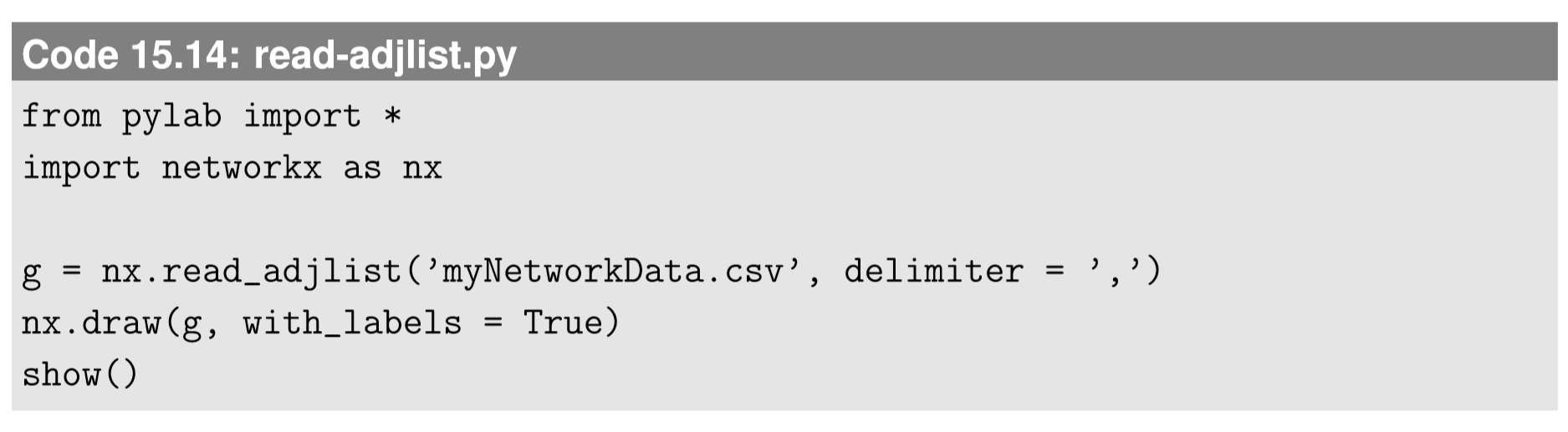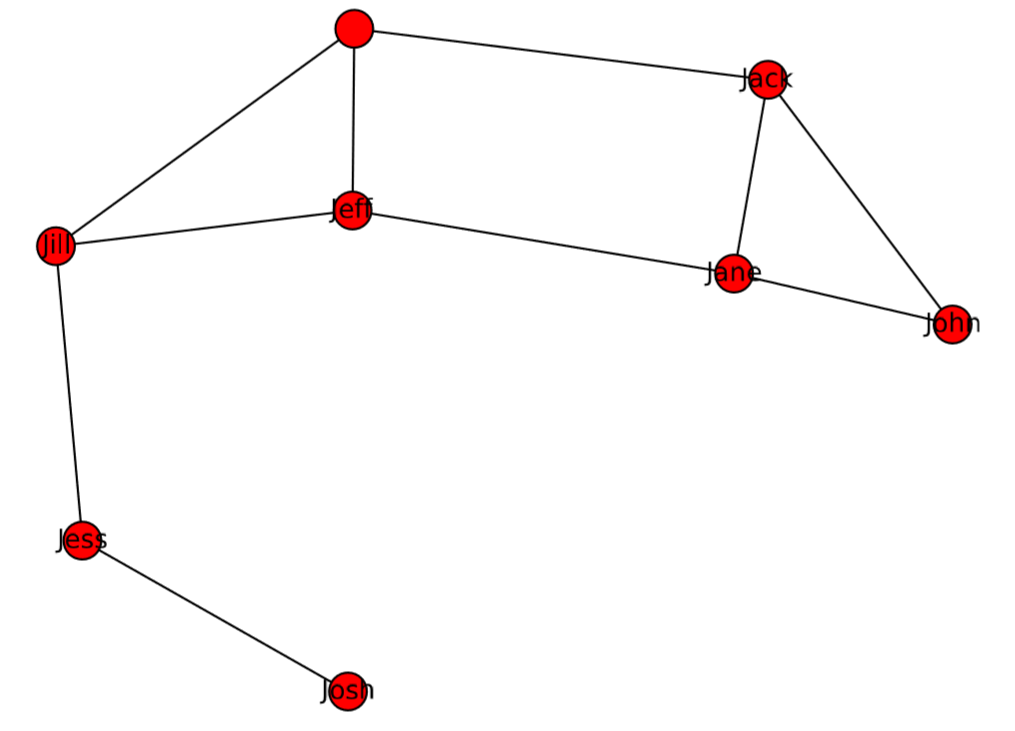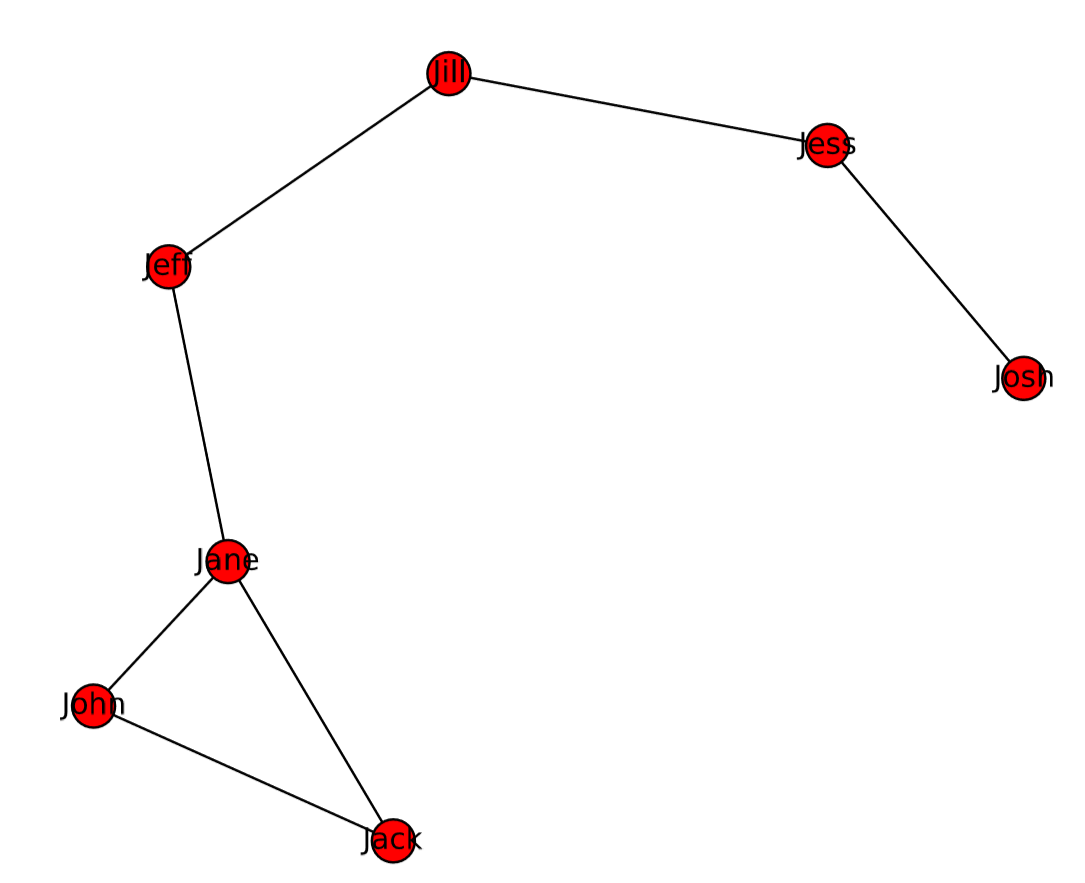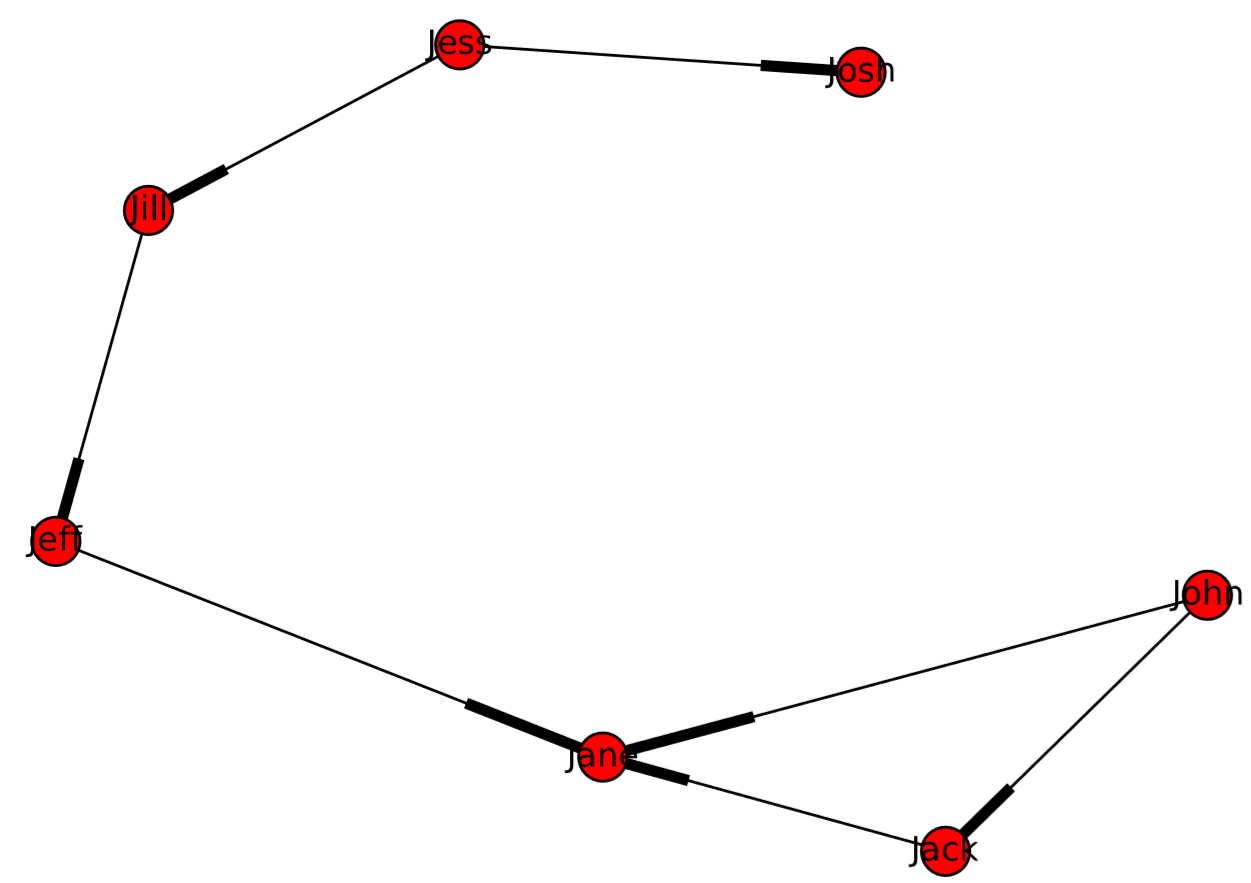$$\newcommand{\id}{\mathrm{id}}$$ $$\newcommand{\Span}{\mathrm{span}}$$ $$\newcommand{\kernel}{\mathrm{null}\,}$$ $$\newcommand{\range}{\mathrm{range}\,}$$ $$\newcommand{\RealPart}{\mathrm{Re}}$$ $$\newcommand{\ImaginaryPart}{\mathrm{Im}}$$ $$\newcommand{\Argument}{\mathrm{Arg}}$$ $$\newcommand{\norm}{\| #1 \|}$$ $$\newcommand{\inner}{\langle #1, #2 \rangle}$$ $$\newcommand{\Span}{\mathrm{span}}$$

# 15.5: Importing/Exporting Network Data

$$\newcommand{\vecs}{\overset { \rightharpoonup} {\mathbf{#1}} }$$ $$\newcommand{\vecd}{\overset{-\!-\!\rightharpoonup}{\vphantom{a}\smash {#1}}}$$$$\newcommand{\id}{\mathrm{id}}$$ $$\newcommand{\Span}{\mathrm{span}}$$ $$\newcommand{\kernel}{\mathrm{null}\,}$$ $$\newcommand{\range}{\mathrm{range}\,}$$ $$\newcommand{\RealPart}{\mathrm{Re}}$$ $$\newcommand{\ImaginaryPart}{\mathrm{Im}}$$ $$\newcommand{\Argument}{\mathrm{Arg}}$$ $$\newcommand{\norm}{\| #1 \|}$$ $$\newcommand{\inner}{\langle #1, #2 \rangle}$$ $$\newcommand{\Span}{\mathrm{span}}$$ $$\newcommand{\id}{\mathrm{id}}$$ $$\newcommand{\Span}{\mathrm{span}}$$ $$\newcommand{\kernel}{\mathrm{null}\,}$$ $$\newcommand{\range}{\mathrm{range}\,}$$ $$\newcommand{\RealPart}{\mathrm{Re}}$$ $$\newcommand{\ImaginaryPart}{\mathrm{Im}}$$ $$\newcommand{\Argument}{\mathrm{Arg}}$$ $$\newcommand{\norm}{\| #1 \|}$$ $$\newcommand{\inner}{\langle #1, #2 \rangle}$$ $$\newcommand{\Span}{\mathrm{span}}$$

In most network studies, researchers need to model and analyze networks that exist in the real world. To do so, we need to learn how to import (and export) network data from outside Python/NetworkX. Fortunately, NetworkX can read and write network data from/to ﬁles in a number of formats2.Figure $$\PageIndex{1}$$: Visual output of Code 15.13, showing examples of drawing options available in NetworkX. The same node positions are used in all panels. The last two examples show the axes because they are generated using different drawing functions. To suppress the axes, use axis(’off’) right after the network drawing.

Let’s work on a simple example. You can create your own adjacency list in a regular spreadsheet (such as Microsoft Excel or Google Spreadsheet) and then save it in a “csv” format ﬁle (Fig. 15.5.2). In this example, the adjacency list says that John is connected to Jane and Jack; Jess is connected to Josh and Jill; and so on.Figure $$\PageIndex{2}$$: Creating an adjacency list in a spreadsheet.

You can read this ﬁle by using the read_adjlist command, as follows:The read_adjlist command generates a network by importing a text ﬁle that lists the names of nodes in the adjacency list format. By default, it considers spaces (’ ’) a separator, so we need to specify the delimiter = ’,’ option to read the CSV comma separated values) ﬁle. Place this Python code in the same folder where the data ﬁle is located, run it, and you will get a result like Fig. 15.5.33.Figure $$\PageIndex{3}$$: Visual output of Code 15.14.

Looks good. No—wait—there is a problem here. For some reason, there is an extra node without a name (at the top of the network in Fig.15.5.3)! What happened? The reason becomes clear if you open the CSV ﬁle in a text editor, which reveals that the ﬁle actually looks like this:Note the commas at the end of the third, fourth, and ﬁfth lines. Many spreadsheet applications tend to insert these extra commas in order to make the number of columns equal for all rows. This is why NetworkX thought there would be another node whose name was “” (blank). You can delete those unnecessary commas in the text editor and save the ﬁle. Or you could modify the visualization code to remove the nameless node before drawing the network. Either way, the updated result is shown in Fig. 15.5.4.Figure $$\PageIndex{4}$$ Visual output of Code 15.14 with a corrected CSV data ﬁle.

If you want to import the data as a directed graph, you can use the create_using option in the read_adjlist command, as follows:The result is shown in Fig.15.5.5, in which arrowheads are indicated by thick line segments.

Another simple function for data importing is read_edgelist. This function reads an edge list, i.e., a list of pairs of nodes, formatted as follows:Figure $$\PageIndex{5}$$: Visual output of Code 15.16.

One useful feature of read_edgelist is that it can import not only edges but also their properties, such as:The third column of this data ﬁle is structured as a Python dictionary, which will be imported as the property of the edge.

Exercise $$\PageIndex{1}$$

Create a data ﬁle of the social network you created in Exercise 15.3.2 using a spreadsheet application, and then read the ﬁle and visualize it using NetworkX. You can use either the adjacency list format or the edge list format.

Exercise $$\PageIndex{2}$$

Import network data of your choice from Mark Newman’s Network Data website: http://www-personal.umich.edu/~mejn/netdata/. The data on this website are all in GML format, so you need to ﬁgure out how to import them into NetworkX. Then visualize the imported network.

Finally, NetworkX also has functions to write network data ﬁles from its graph objects, such as write_adjlist and write_edgelist. Their usage is very straightforward. Here is an example:Then a new text ﬁle named ’complete-graph.txt’ will appear in the same folder, which looks like this:Note that the adjacency list is optimized so that there is no redundant information included in this output. For example, node 4 is connected to 0, 1, 2, and 3, but this information is not included in the last line because it was already represented in the preceding lines.

Exercise $$\PageIndex{3}$$

Write the network data of Zachary’s Karate Club graph into a ﬁle in each of the following formats:

2For more details, see https://networkx.github.io/documenta...ence/readwrite. html.